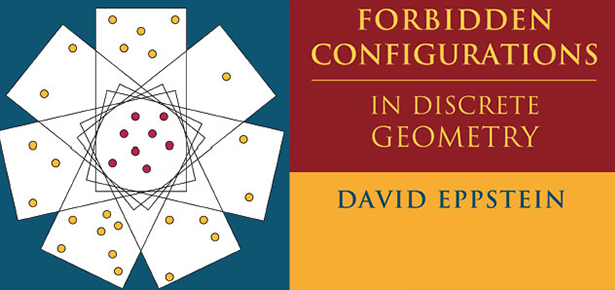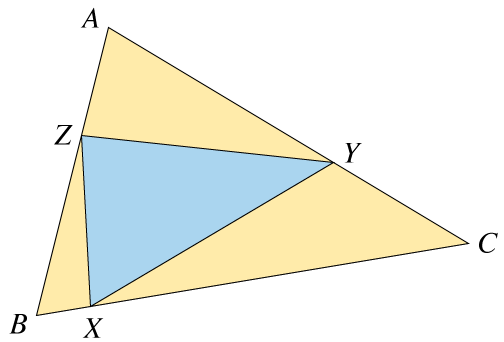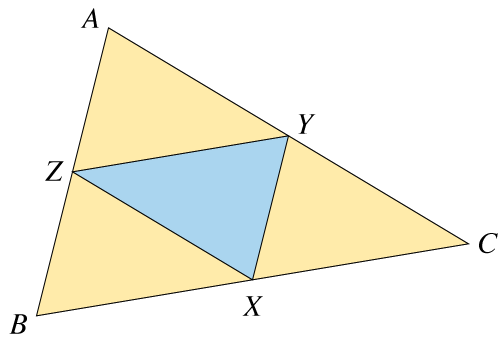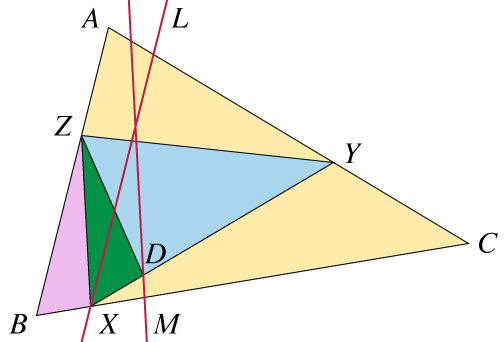Products and services

# Fifteen Eighty Four

13
Apr
2018

## Esther Szekeres on triangle inequalities

David EppsteinEsther Klein (later Esther Szekeres) famously observed that five points in the plane with no three in line must contain the vertices of a convex quadrilateral. Similarly, nine points in the plane with no three in line must contain the vertices of a convex pentagon, and more generally for every n there exists a larger number N such that every N points in the plane with no three in line must contain the vertices of a convex n-gon. The “happy ending problem” asks for a more precise relation between n and N; it is still the subject of ongoing research, including a 2016 breakthrough by Andrew Suk, and is one of the topics covered in my about-to-be-published book Forbidden Configurations in Discrete Geometry.

After this incident, the particulars of Klein’s life become difficult to separate from those of her eventual husband, George Szekeres (the one who proved the more general statement about n-gons). Neither started out as mathematicians. Because of the restrictions placed on Jews in Hungary in the late 1920s, only two students from Szekeres’s school could study science or mathematics at the university in Budapest; Márta Svéd took the mathematics position, so Klein necessarily studied physics instead. George studied chemical engineering, motivated by his family’s leather business. The two became refugees in Shanghai, and then after the end of World War II moved to Adelaide, where they shared an apartment with Márta Svéd and her family. George became a university mathematics lecturer and Esther raised their children while working as a mathematics tutor. In 1964, the family moved to Sydney. Esther became one of the first mathematicians at the newly-founded Macquarie University, where she is “fondly remembered as a gifted and inspiring tutor”; Macquarie gave her an honorary doctorate in 1990. She and her husband died within hours of each other, in 2005.

Their joint Sydney Morning Herald obituary writes of Esther that “The mathematical love of her life was always geometry, in which she outshone George.” So with this as background, I was interested to learn more about some of her work in geometry. I found a paper, “Einfache Beweise zweier Dreieckssätze”, that she published in 1967 in the journal Elemente der Mathematik (in German, despite being a Hungarian in Australia). The title promises two theorems about triangles, both of which concern what happens when you inscribe a triangle XYZ into a larger triangle ABC (with X opposite A, etc), dividing ABC into four smaller triangles.Szekeres’s two theorems are that the area and perimeter of the central triangle XYZ are at least equal to the minimum area or perimeter among the three surrounding triangles. It’s possible for XYZ to be one of the smallest triangles, but this can only happen when XYZ has equal area or perimeter to another of the four small triangles; it can never be the unique smallest one. For instance, when XYZ is the medial triangle of ABC, all four smaller triangles are congruent to each other (and similar to the big triangle).The theorems themselves are not original to Szekeres, and her paper details their history of publication and solution in various mathematical problem columns. The perimeter inequality is also connected with a classical piece of geometry, Fagnano’s problem of finding an inscribed triangle XYZ of the minimum possible perimeter.

Stripped of some unnecessary detail, her proof of the area theorem is simple and elegant. Suppose that BX:BC is the smallest of the six ratios into which the three points XYZ divide the sides of the triangle; the other five cases are symmetric. Draw two additional lines, L through X parallel to AB, and M parallel to XZ but twice as far from B. Then it follows from the choice of BX:BC as the smallest ratio that Y lies on the segment of AC on the far side of B from L, and that M separates X from this segment.So if we place a point D at the intersection of line M and segment XY, we have

area(XZB) = area(XZD) ≤ area(XYZ),

where the left equality relates two triangles with the same base XZ and equal heights, and the right inequality is containment of one triangle in the other.Most of Szekeres’s other publications were in mathematical problem columns, and included similar styles of reasoning applied to other geometry problems. Beyond geometry, the subjects of her research included arithmetic combinatorics and graph theory. Still, it is clear that it is in geometry, and in particular in the problem of convex polygons in point sets, where she made her most far-reaching contribution to mathematics. Her problem became foundational for two major fields, discrete geometry and Ramsey theory, and has led to a huge body of research by other mathematicians.

For other posts by David Eppstein, visit his blog. Also make sure to find out more about Forbidden Configurations in Discrete Geometry